Vibrations Index Page

Natural Frequencies to Traverse Vibrations

Introduction

The notes below relate to transverse vibrations of beams and the calculation of the natural frequency.    These notes only relate to the lowest natural frequency.    There are of course an infinite number of natural frequencies but generally only the lowest one has an engineering relevance   This derivation of the equations is provided using two methods.    An example to determine the natural frequency of a simply supported uniform beam is completed using both methods.    The notes also show the relationship between the natural frequency of vibration of a shaft and the whirling speed of a shaft.    Whirling is a result of resonance when the shaft rotates at the same speed as one of the shafts natural frequencies of transverse vibration.

Nomenclature

The units below relate to application of the equations using SI metric values

 L = Length (m) m = mass/Unit length (kg/m) Ma = Mass of Disk (kg) E = Youngs Modulus (N/m2 f = frequency of vibration /whirling frequency (Hz= cycles/s) g = acceleration due to gravity (m/s 2) I = Area Moment of Inertia (m4) ω = angular velocity (rads/s)

Transverse Vibration - Short Derivation of Natural Frequency.

Referring to Free vibrations it is clear that a beam, when deflected, will tend to vibrate.    If there was no internal damping the beam would continue to vibrate at its natural frequency.    This frequency relates directly to the inertial force resulting from the mass in motion and the potential energy stored in the beam due to its elasticity.

Consider a thin beam of with a mass/unit length of = m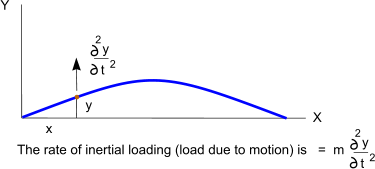For a beam subject to inertial vibrating motion the force w is the elastic force / unit length causing the beam to deflect from its static geometry.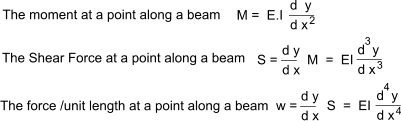Assuming that the uniform beam is loaded only as a result of inertial forces.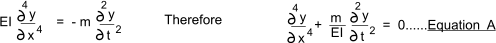Assuming the motion of any point is a simple harmonic vibration. Then let let.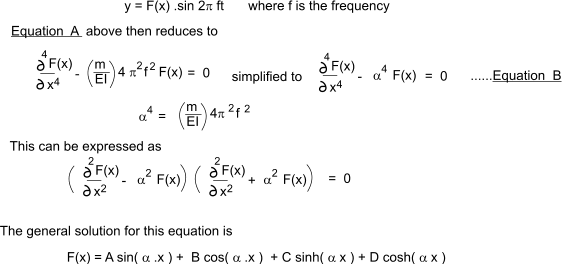Differentiating this equation once and then again results in the following equations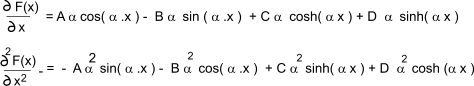The above equations can be used to arrive at the natural frequency of a uniform beam under different support scenarios.

Example - A simply supported beam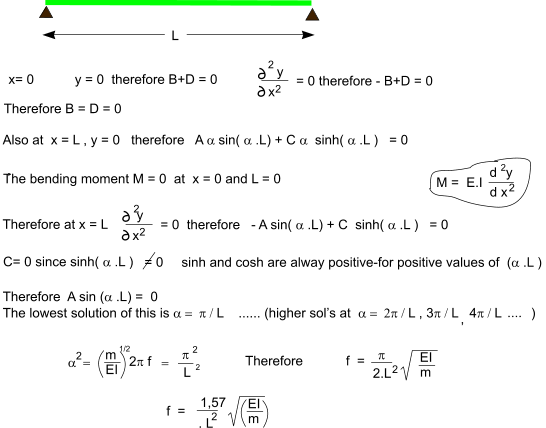Transverse Vibration - Short Derivation of Natural Frequency. Using energy method.

This is a more approximate method as it assumes that a vibrating beam assumes the shape similar to that of a horizontal static deflection curve .    It has been proved that this is acceptably accurate for practical applications.

The beam vibration takes the shape .

Y = F(x) is the static beam shape and k = a constant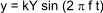In the extreme position (sin ( 2 π f t) = 1)     All of the enrgy is in the form of potential stored energy and y = kY. the equivalent static load to deflect the beam to this position is k m g hence the total strain energy is .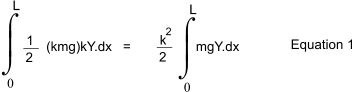In the mid position all of the energy is kinetic and the velocity is given by .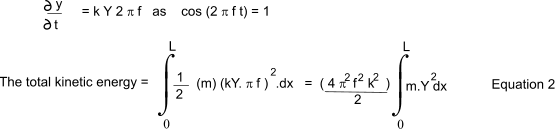Combining Equations 1 and 2 results in .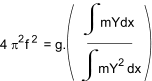Repeated example - A simply supported beam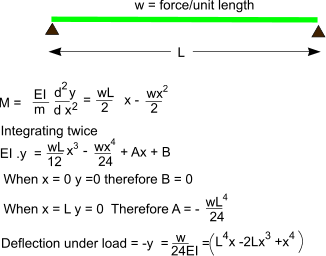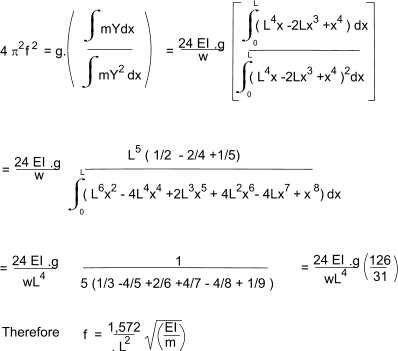It is clear that both methods yields a similar result.    The second method is slightly less accurate.    It must be recognised that for real beam with significant section sizes these methods, based only on transvers bending deflections, may only produce approximate results.    Larger beams are also affected by axial and shear stresses.

Whirling of Shafts.

In practice the centre of the mass of a shaft does not exactly correspond to the axis of rotation of the shaft.     This may be due to out of straightness, vibration , out of roundess etc.     Hence as a shaft is rotated there are centrifugal forces generated resulting in bending moments which tend to deflect the shaft, resulting in increasing centrifugal forces.    The transverse forces are proportional to the rotation speed2 and the deflection.    The direction of the centrifugal force is related to the angle of rotation.    The forces are resisted by the internal elastic forces in the shaft (its stiffness) .    When the point is reached when the rotation speed equals a natural frequency of the shaft.    The out of balance cyclic motion drives the vibration of the shaft and it begins to whirl and the shaft is in an unstable condition.    High stresses and vibrations will result if the shaft is maintained at this speed.    If the speed is further increased the shaft deflection will increase but the instability will cease.     Shaft whirling only occurs when the cyclic centrifugal force = a shaft natural frequency.    Whirling is an example of forced vibraton

Whirling of a uniform shaft.

If the mass of a shaft /unit length is m and y is the deflection of the shaft at any point for an angular velocity of ω the transverse centrifugal force = -myω 2.

When the shaft commences to "whirl" the centrifugal force is just balanced by the stiffness of the beam. i.e. EI.( d4y / dx4 )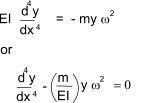If the rotational speed of the shaft is "n" eg. revs/sec. (n 2.π = ω) the the equation is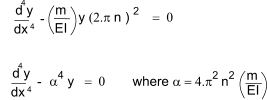This is a very similar equation to that for the transverse vibration of a shaft (Equation B above) and the determination of n is the same as the determination of the shaft natual frequency f.

Whirling of a eccentrically mounted mass.

Consider a Mass Ma mounted on a shaft with its centre of gravity displaced e from the shaft rotation axis.     When the shaft is rotated . The shaft will deflect a distance y .

The stiffnes of the shaft k is defined as the restoring force per unit deflection.    As in the case of sping the Sqrt (k/Ma ) = ωn 2... ωn being the resonant (natural) frequency. of the beam.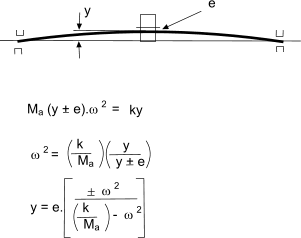It is clear that the deflection y tends to infinity when ω -> Sqrt (k/Ma )= ωn

 Links to shaft Vibrations Basic Theory of Vibrations...Chaptertwo of a text book by RE Blake
Vibrations Index Page